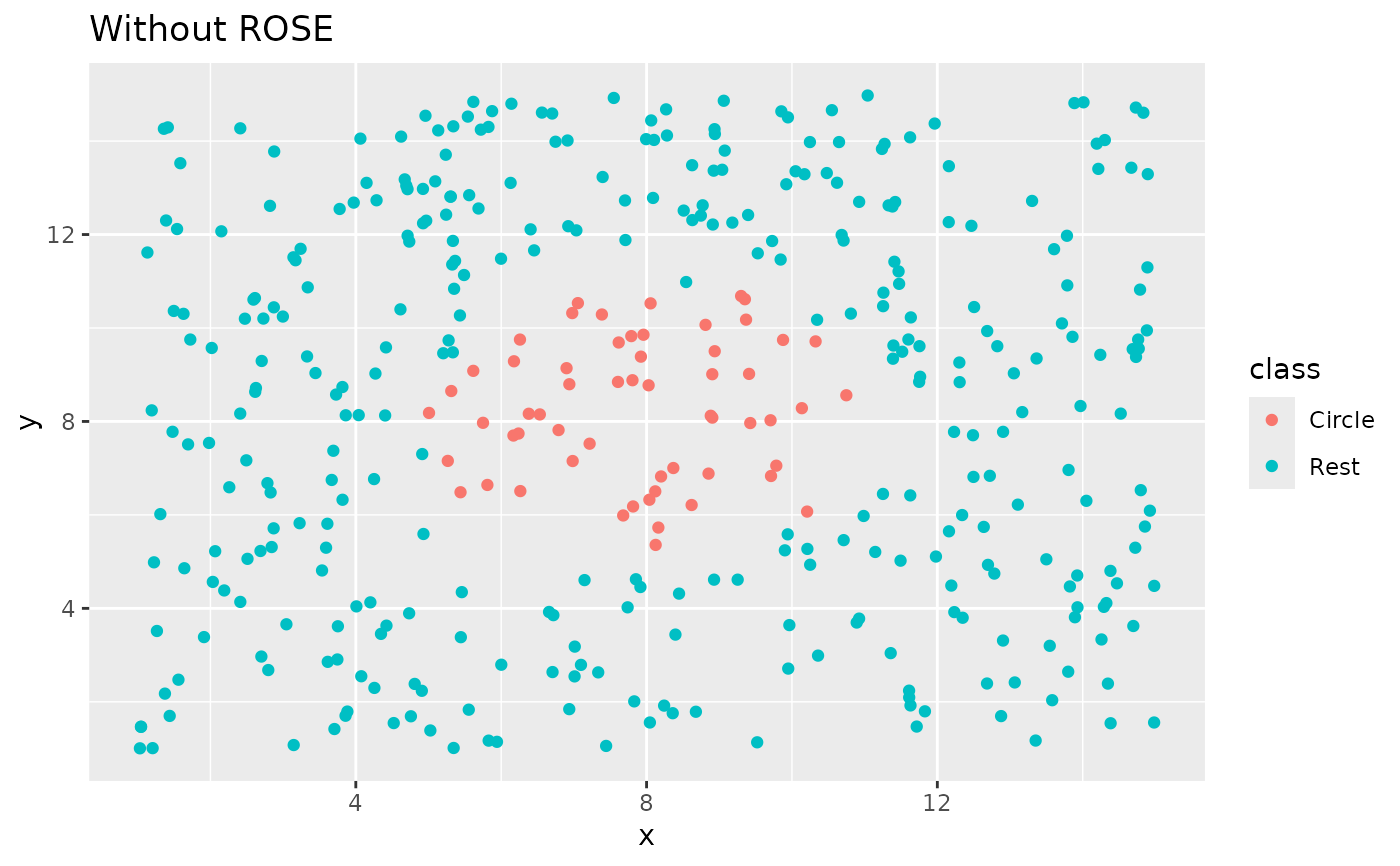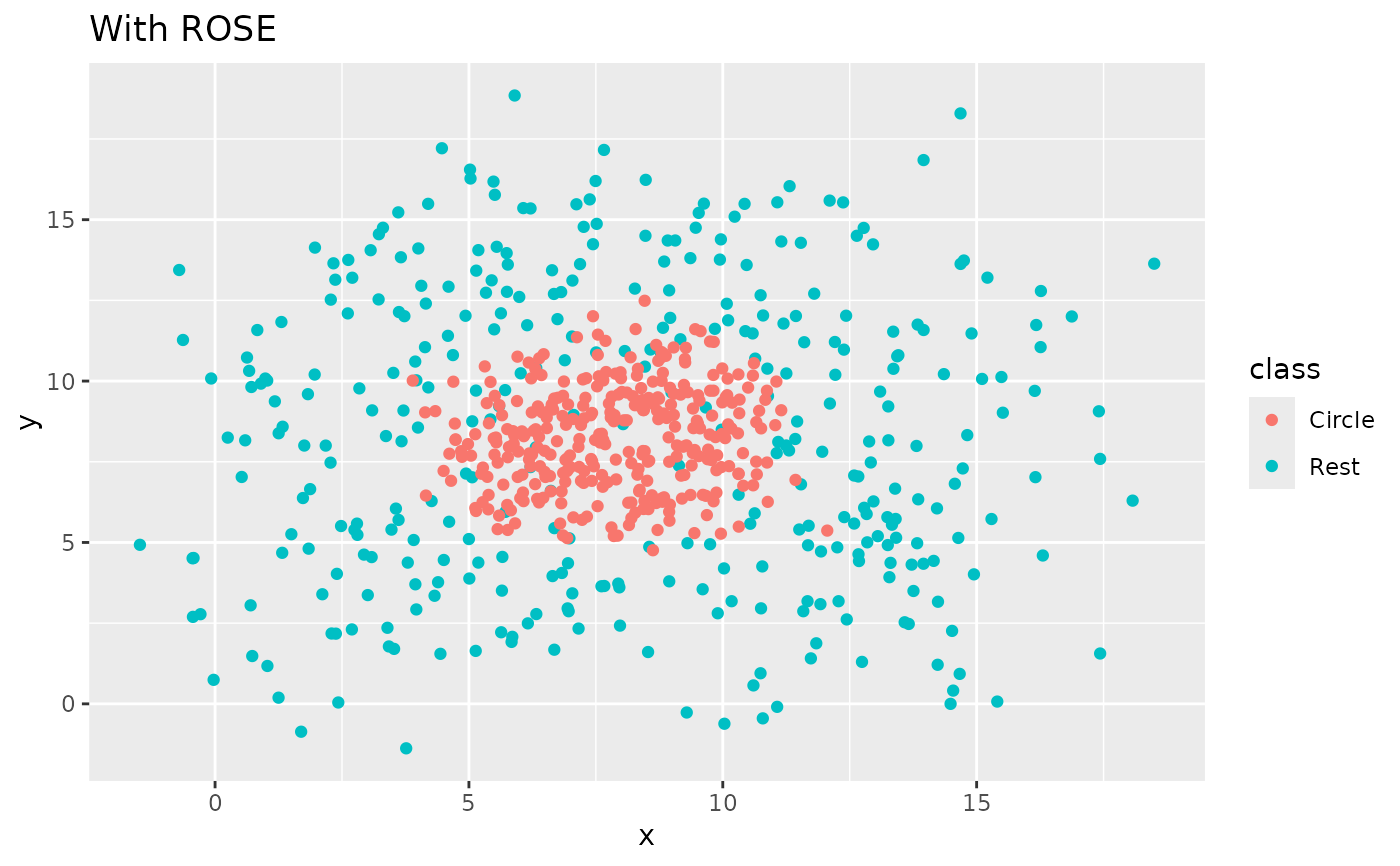step_rose creates a specification of a recipe step that generates sample of synthetic data by enlarging the features space of minority and majority class example. Using ROSE::ROSE().

step_rose(
recipe,
...,
role = NA,
trained = FALSE,
column = NULL,
over_ratio = 1,
minority_prop = 0.5,
minority_smoothness = 1,
majority_smoothness = 1,
skip = TRUE,
seed = sample.int(10^5, 1),
id = rand_id("rose")
)

# S3 method for step_rose
tidy(x, ...)

## Arguments

recipe A recipe object. The step will be added to the sequence of operations for this recipe. One or more selector functions to choose which variable is used to sample the data. See selections() for more details. The selection should result in single factor variable. For the tidy method, these are not currently used. Not used by this step since no new variables are created. A logical to indicate if the quantities for preprocessing have been estimated. A character string of the variable name that will be populated (eventually) by the ... selectors. A numeric value for the ratio of the majority-to-minority frequencies. The default value (1) means that all other levels are sampled up to have the same frequency as the most occurring level. A value of 0.5 would mean that the minority levels will have (at most) (approximately) half as many rows than the majority level. A numeric. Determines the of over-sampling of the minority class. Defaults to 0.5. A numeric. Shrink factor to be multiplied by the smoothing parameters to estimate the conditional kernel density of the minority class. Defaults to 1. A numeric. Shrink factor to be multiplied by the smoothing parameters to estimate the conditional kernel density of the majority class. Defaults to 1. A logical. Should the step be skipped when the recipe is baked by bake.recipe()? While all operations are baked when prep.recipe() is run, some operations may not be able to be conducted on new data (e.g. processing the outcome variable(s)). Care should be taken when using skip = TRUE as it may affect the computations for subsequent operations An integer that will be used as the seed when rose-ing. A character string that is unique to this step to identify it. A step_rose object.

## Value

An updated version of recipe with the new step added to the sequence of existing steps (if any). For the tidy method, a tibble with columns terms which is the variable used to sample.

## Details

The factor variable used to balance around must only have 2 levels.

The ROSE algorithm works by selecting an observation belonging to class k and generates new examples in its neighborhood is determined by some matrix H_k. Smaller values of these arguments have the effect of shrinking the entries of the corresponding smoothing matrix H_k, Shrinking would be a cautious choice if there is a concern that excessively large neighborhoods could lead to blur the boundaries between the regions of the feature space associated with each class.

All columns in the data are sampled and returned by juice() and bake().

All columns used in this step must be numeric.

When used in modeling, users should strongly consider using the option skip = TRUE so that the extra sampling is not conducted outside of the training set.

Lunardon, N., Menardi, G., and Torelli, N. (2014). ROSE: a Package for Binary Imbalanced Learning. R Jorunal, 6:82–92.

Menardi, G. and Torelli, N. (2014). Training and assessing classification rules with imbalanced data. Data Mining and Knowledge Discovery, 28:92–122.

## Examples

library(recipes)
library(modeldata)
data(okc)

sort(table(okc$Class, useNA = "always")) #> #> <NA> stem other #> 0 9539 50316 ds_rec <- recipe(Class ~ age + height, data = okc) %>% step_rose(Class) %>% prep() sort(table(bake(ds_rec, new_data = NULL)$Class, useNA = "always"))
#>
#>  <NA>  stem other
#>     0 50255 50377
# since skip defaults to TRUE, baking the step has no effect
baked_okc <- bake(ds_rec, new_data = okc)
table(baked_okc$Class, useNA = "always") #> #> stem other <NA> #> 9539 50316 0 ds_rec2 <- recipe(Class ~ age + height, data = okc) %>% step_rose(Class, minority_prop = 0.3) %>% prep() table(bake(ds_rec2, new_data = NULL)$Class, useNA = "always")
#>
#>  stem other  <NA>
#> 30452 70180     0
library(ggplot2)

ggplot(circle_example, aes(x, y, color = class)) +
geom_point() +
labs(title = "Without ROSE")recipe(class ~ ., data = circle_example) %>%
step_rose(class) %>%
prep() %>%
bake(new_data = NULL) %>%
ggplot(aes(x, y, color = class)) +
geom_point() +
labs(title = "With ROSE")